### Home > CCA2 > Chapter 8 > Lesson 8.1.3 > Problem8-60

8-60.
1.Without using a calculator, find the solution to each equation. Homework Help ✎

1. 2x = 17

2. log3(x + 1) = 5

3. log3(3x) = 4

4. 4log4(x) = 7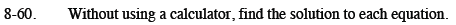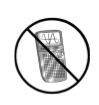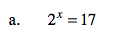Start by taking the log of both sides.

$x=\frac{\text{log}17}{\text{log}2}$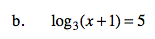x = 242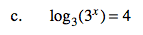x = 4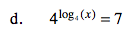x = 7